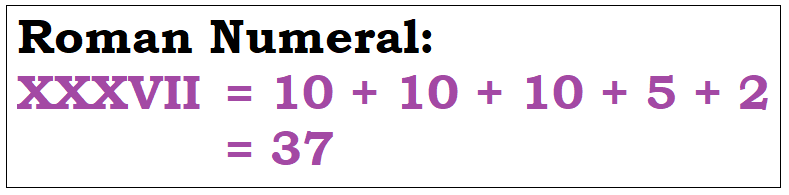Checkout JEE MAINS 2022 Question Paper Analysis : Checkout JEE MAINS 2022 Question Paper Analysis :

# XXXVII Roman Numerals

What are Roman Numerals?

Roman Numerals are a special kind of numerical notations that were first used by the Romans. This is an additive and subtractive system, where letters are used to indicate specific base numbers and arbitrary numbers in the number system and are denoted using a different combination of letters or symbols. Numbers in this system are represented by combinations of a mixed or specific collection of letters from the Latin alphabet. The modern method uses seven characters; each of these characters holds a fixed integer value as given below:

 Character (or Letter) I V X L C D M Numerical Value 1 5 10 50 100 500 1000

## XXXVII Value in Roman Numerals

In roman numerals, the value of XXXVII can be found using the addition method. Here, first we need to write the numerical value of each character or letter. Then, add these numbers to get the value of XXXVII. This addition can be done in many ways as explained below:Method 1: In this method, write the numerical value of each letter and add them to get the result.

XXXVII = 10 + 10 + 10 + 5 + 1 + 1 = 37

Method 2: In this method, the numerical values of groups of letters are considered for addition such as:

XXXVII = (XXX) + (VII) = 30 + 7 = 37

Alternatively,

XXXVII = (XXX) + (V) + (II) = 30 + 5 + 2 = 37

Therefore,

 XXXVII = 37

The above methods are used to find the numerical value of all the roman numerals to a possible extent.

### How to convert Roman numerals to Numbers?

Before going to conversion of roman numbers to numbers, first understand the representation of this system of numbers in detail. Consider the below sequence:

I, II, III, IV, V, VI, VII, VIII, IX, X, XI, XII, XIII, XIV, XV, XVI, XVII, XVIII, XIX, XX

From this, we can observe that the notations IV and IX can be read, respectively as “one less than five, i.e. 4” and “one less than ten, i.e. 9”. Also, VI and XI can be read, respectively as “one more than 5, i.e. 6” and “one more than 10, i.e. 11”. In this way, the large numbers can also be represented with the specific notations.

The below table shows the convention rules that will help in writing numbers in roman numerals accurately.

 Units Tens Hundreds Thousands 1 I X C M 2 II XX CC MM 3 III XXX CCC MMM 4 IV XL CD 5 V L D 6 VI LX DC 7 VII LXX DCC 8 VIII LXXX DCCC 9 IX XC CM

## Video Lesson on Roman Numerals### Numbers nearer to XXXVII

The numbers which are close to, less than and greater than XXXVII are given below:

XXX = 10 + 10 + 10 = 30

XXXI = 30 + 1 = 31

XXXII = 30 + 1 + 1 = 32

XXXIII = 30 + 1 + 1 + 1 = 33

XXXIV = 30 + 4 = 34

XXXV = 30 + 5 = 35

XXXVI = 30 + 6 = 36

XXXVII = 30 + 7 = 37

XXXVIII = 30 + 8 = 38

XXXIX = 30 + 9 = 39

XL = 40

XLI = 40 + 1 = 41

XLII = 40 + 1 + 1 = 42

XLIII = 40 + 1 + 1 + 1 = 43

Test your Knowledge on XXXVII Roman Numerals